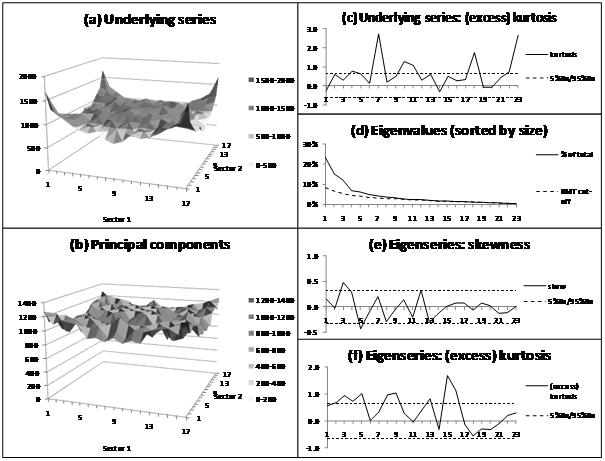/

### Extreme Events – Figure 3.19: Impact of adjusting every series simultaneously by recent past cross-sectional volatility

Sub-charts:

(a)    Number of observations in each fractile-fractile pairing

(b)   Number of observations for each principal component pairing (weighted by eigenvalue size)

(c)    (Excess) kurtosis of each underlying return series

(d)   Eigenvalues (sorted by size)

(e)   Skewness of each eigenseries (sorted in order of decreasing eigenvalue size)

(f)     (Excess) kurtosis of each eigenseries (sorted in order of decreasing eigenvalue size)

SmartChart version:

Not available

Variant used in book:Source: Nematrian, Thomson Datastream. Copyright (c) Nematrian (2010)